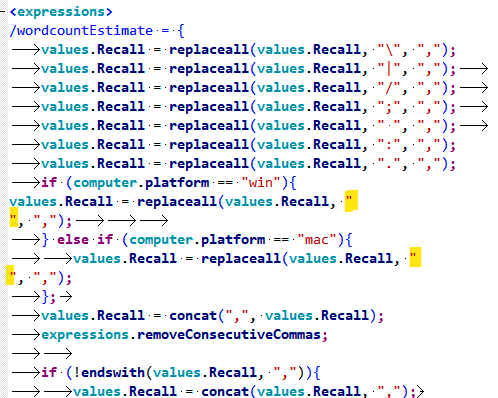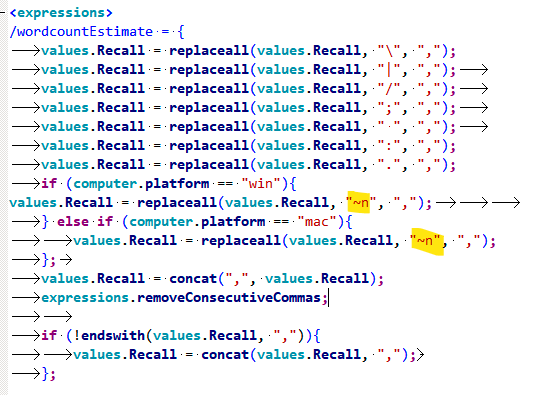Disable/remove next button for period of time

Disable/remove next button for period of time
 Author Message dproostposted Last Month ANSWER HOTGroup: Forum Members Posts: 7, Visits: 62 Dear all In my experiment, I want to provide participants a textbox in which they have to fill in all words they remember from previously seen word lists. The total duration they get to do this task, is 5 minutes. Everything works fine, apart from the fact that participants shouldn't be allowed to press 'continue' before the 5 minutes are over. In a similar topic on the forum, I found that you could make use of 'isvalidresponse' to make sure participants can't advance. However, this only seems to work for Inquisit 5 and not for version 6. Or am I missing something else? Thank you in advance for your help! Here, I provide some code for clarification:/ items = ("Recall as many of the words from all studied lists as you can. You can either start each word on a new line, or add spaces or commas in between words. Your remaining time is: You cannot press the 'continue' button before the 5 minutes are over.")/ position = (50%, 10%)/ fontstyle = ("Arial", 3%, true, false, false, false, 5, 1)/ size = (90%, 10%)/ stimulusframes = [1 = recallInstructions]/ pretrialpause = parameters.recallDelay/ ontrialbegin = [    values.Recall = "";    values.countCorrect_neutral = 0;    values.countCorrect_low = 0;    values.countCorrect_high = 0;    values.countCritical_neutral = 0;    values.countCritical_low = 0;    values.countCritical_high = 0;    values.phase = "recall";]/ size = (20%, 30%)/ multiline = true/ charlimit = 5000/ position = (50%, 53%)/ isvalidresponse = [openended.recall_overallgroup1.latency >= 300000].../ timeout = parameters.maxRecallDuration/ preinstructions = (recallPhase)/ bgstim = (clock.timerrecall)/ onblockbegin = [    values.RecallWordEstimate = "";    values.Recall = "";    values.nr_otherRecalledWords = "";    values.countCorrect_neutral = 0;    values.countCorrect_low = 0;    values.countCorrect_high = 0;    values.countCritical_neutral = 0;    values.countCritical_low = 0;    values.countCritical_high = 0;    values.intrusionOther = "";    values.lure = "";]/ trials = [1 = recall_overallgroup1]/ onblockend = [    values.nextList = "";] Daveposted Last Month ANSWERGroup: Administrators Posts: 11K, Visits: 77K +x dproost - 12/7/2021Dear all In my experiment, I want to provide participants a textbox in which they have to fill in all words they remember from previously seen word lists. The total duration they get to do this task, is 5 minutes. Everything works fine, apart from the fact that participants shouldn't be allowed to press 'continue' before the 5 minutes are over. In a similar topic on the forum, I found that you could make use of 'isvalidresponse' to make sure participants can't advance. However, this only seems to work for Inquisit 5 and not for version 6. Or am I missing something else? Thank you in advance for your help! Here, I provide some code for clarification:/ items = ("Recall as many of the words from all studied lists as you can. You can either start each word on a new line, or add spaces or commas in between words. Your remaining time is: You cannot press the 'continue' button before the 5 minutes are over.")/ position = (50%, 10%)/ fontstyle = ("Arial", 3%, true, false, false, false, 5, 1)/ size = (90%, 10%)/ stimulusframes = [1 = recallInstructions]/ pretrialpause = parameters.recallDelay/ ontrialbegin = [    values.Recall = "";    values.countCorrect_neutral = 0;    values.countCorrect_low = 0;    values.countCorrect_high = 0;    values.countCritical_neutral = 0;    values.countCritical_low = 0;    values.countCritical_high = 0;    values.phase = "recall";]/ size = (20%, 30%)/ multiline = true/ charlimit = 5000/ position = (50%, 53%)/ isvalidresponse = [openended.recall_overallgroup1.latency >= 300000].../ timeout = parameters.maxRecallDuration/ preinstructions = (recallPhase)/ bgstim = (clock.timerrecall)/ onblockbegin = [    values.RecallWordEstimate = "";    values.Recall = "";    values.nr_otherRecalledWords = "";    values.countCorrect_neutral = 0;    values.countCorrect_low = 0;    values.countCorrect_high = 0;    values.countCritical_neutral = 0;    values.countCritical_low = 0;    values.countCritical_high = 0;    values.intrusionOther = "";    values.lure = "";]/ trials = [1 = recall_overallgroup1]/ onblockend = [    values.nextList = "";]The easy fix is to add /required = true to the element. dproostposted Last Month ANSWERGroup: Forum Members Posts: 7, Visits: 62 +x Dave - 12/7/2021+x dproost - 12/7/2021Dear all In my experiment, I want to provide participants a textbox in which they have to fill in all words they remember from previously seen word lists. The total duration they get to do this task, is 5 minutes. Everything works fine, apart from the fact that participants shouldn't be allowed to press 'continue' before the 5 minutes are over. In a similar topic on the forum, I found that you could make use of 'isvalidresponse' to make sure participants can't advance. However, this only seems to work for Inquisit 5 and not for version 6. Or am I missing something else? Thank you in advance for your help! Here, I provide some code for clarification:/ items = ("Recall as many of the words from all studied lists as you can. You can either start each word on a new line, or add spaces or commas in between words. Your remaining time is: You cannot press the 'continue' button before the 5 minutes are over.")/ position = (50%, 10%)/ fontstyle = ("Arial", 3%, true, false, false, false, 5, 1)/ size = (90%, 10%)/ stimulusframes = [1 = recallInstructions]/ pretrialpause = parameters.recallDelay/ ontrialbegin = [    values.Recall = "";    values.countCorrect_neutral = 0;    values.countCorrect_low = 0;    values.countCorrect_high = 0;    values.countCritical_neutral = 0;    values.countCritical_low = 0;    values.countCritical_high = 0;    values.phase = "recall";]/ size = (20%, 30%)/ multiline = true/ charlimit = 5000/ position = (50%, 53%)/ isvalidresponse = [openended.recall_overallgroup1.latency >= 300000].../ timeout = parameters.maxRecallDuration/ preinstructions = (recallPhase)/ bgstim = (clock.timerrecall)/ onblockbegin = [    values.RecallWordEstimate = "";    values.Recall = "";    values.nr_otherRecalledWords = "";    values.countCorrect_neutral = 0;    values.countCorrect_low = 0;    values.countCorrect_high = 0;    values.countCritical_neutral = 0;    values.countCritical_low = 0;    values.countCritical_high = 0;    values.intrusionOther = "";    values.lure = "";]/ trials = [1 = recall_overallgroup1]/ onblockend = [    values.nextList = "";]The easy fix is to add /required = true to the element.Thank you for your response, it works now! I still have one additional question. I don't know whether it's because of this little change, but now, our code which we used to be able to count the number of words automatically doesn't seem to work anymore. Could you think of a possible explanation or solution for this problem? Thanks in advance!This is the code we use to separate each word with a comma and then count the total number of words: /wordcountEstimate = {    values.Recall = replaceall(values.Recall, "\", ",");    values.Recall = replaceall(values.Recall, "|", ",");        values.Recall = replaceall(values.Recall, "/", ",");        values.Recall = replaceall(values.Recall, ";", ",");        values.Recall = replaceall(values.Recall, " ", ",");        values.Recall = replaceall(values.Recall, ":", ",");    values.Recall = replaceall(values.Recall, ".", ",");    if (computer.platform == "win"){values.Recall = replaceall(values.Recall, "", ",");                } else if (computer.platform == "mac"){        values.Recall = replaceall(values.Recall, "", ",");    };        values.Recall = concat(",", values.Recall);    expressions.removeConsecutiveCommas;            if (!endswith(values.Recall, ",")){        values.Recall = concat(values.Recall, ",");        };    values.RecallLength = length(values.Recall);    values.reducedRecall = replaceall(values.Recall, ",", "");        values.RecallWordEstimate= values.RecallLength - length(values.reducedRecall) - 1;}/removeConsecutiveCommas = {    values.Recall = replaceall(values.Recall, ",,", ",");    if (contains(values.Recall, ",,")){        expressions.removeConsecutiveCommas;    };} Daveposted Last Month ANSWERGroup: Administrators Posts: 11K, Visits: 77K +x dproost - 12/8/2021+x Dave - 12/7/2021+x dproost - 12/7/2021Dear all In my experiment, I want to provide participants a textbox in which they have to fill in all words they remember from previously seen word lists. The total duration they get to do this task, is 5 minutes. Everything works fine, apart from the fact that participants shouldn't be allowed to press 'continue' before the 5 minutes are over. In a similar topic on the forum, I found that you could make use of 'isvalidresponse' to make sure participants can't advance. However, this only seems to work for Inquisit 5 and not for version 6. Or am I missing something else? Thank you in advance for your help! Here, I provide some code for clarification:/ items = ("Recall as many of the words from all studied lists as you can. You can either start each word on a new line, or add spaces or commas in between words. Your remaining time is: You cannot press the 'continue' button before the 5 minutes are over.")/ position = (50%, 10%)/ fontstyle = ("Arial", 3%, true, false, false, false, 5, 1)/ size = (90%, 10%)/ stimulusframes = [1 = recallInstructions]/ pretrialpause = parameters.recallDelay/ ontrialbegin = [    values.Recall = "";    values.countCorrect_neutral = 0;    values.countCorrect_low = 0;    values.countCorrect_high = 0;    values.countCritical_neutral = 0;    values.countCritical_low = 0;    values.countCritical_high = 0;    values.phase = "recall";]/ size = (20%, 30%)/ multiline = true/ charlimit = 5000/ position = (50%, 53%)/ isvalidresponse = [openended.recall_overallgroup1.latency >= 300000].../ timeout = parameters.maxRecallDuration/ preinstructions = (recallPhase)/ bgstim = (clock.timerrecall)/ onblockbegin = [    values.RecallWordEstimate = "";    values.Recall = "";    values.nr_otherRecalledWords = "";    values.countCorrect_neutral = 0;    values.countCorrect_low = 0;    values.countCorrect_high = 0;    values.countCritical_neutral = 0;    values.countCritical_low = 0;    values.countCritical_high = 0;    values.intrusionOther = "";    values.lure = "";]/ trials = [1 = recall_overallgroup1]/ onblockend = [    values.nextList = "";]The easy fix is to add /required = true to the element.Thank you for your response, it works now! I still have one additional question. I don't know whether it's because of this little change, but now, our code which we used to be able to count the number of words automatically doesn't seem to work anymore. Could you think of a possible explanation or solution for this problem? Thanks in advance!This is the code we use to separate each word with a comma and then count the total number of words: /wordcountEstimate = {    values.Recall = replaceall(values.Recall, "\", ",");    values.Recall = replaceall(values.Recall, "|", ",");        values.Recall = replaceall(values.Recall, "/", ",");        values.Recall = replaceall(values.Recall, ";", ",");        values.Recall = replaceall(values.Recall, " ", ",");        values.Recall = replaceall(values.Recall, ":", ",");    values.Recall = replaceall(values.Recall, ".", ",");    if (computer.platform == "win"){values.Recall = replaceall(values.Recall, "", ",");                } else if (computer.platform == "mac"){        values.Recall = replaceall(values.Recall, "", ",");    };        values.Recall = concat(",", values.Recall);    expressions.removeConsecutiveCommas;            if (!endswith(values.Recall, ",")){        values.Recall = concat(values.Recall, ",");        };    values.RecallLength = length(values.Recall);    values.reducedRecall = replaceall(values.Recall, ",", "");        values.RecallWordEstimate= values.RecallLength - length(values.reducedRecall) - 1;}/removeConsecutiveCommas = {    values.Recall = replaceall(values.Recall, ",,", ",");    if (contains(values.Recall, ",,")){        expressions.removeConsecutiveCommas;    };}    Give me some code I can actually run and examine, please, not an isolated snippet. dproostposted Last Month ANSWERGroup: Forum Members Posts: 7, Visits: 62 Of course, you can find the script in attachment! You'll notice that the code isn't really concise, but I'll hope you can make something out of it. In the extensive part of "openended recall_overallgroup1/2", starting from " / ontrialbegin", we check for all possible right responses (words that occur in the lists). The goal was that the answers are separated by commas automatically. Attachmentsscript_06_12_Inquisit6-consent!.iqx (62 views, 153.00 KB) Daveposted Last Month ANSWERGroup: Administrators Posts: 11K, Visits: 77K +x dproost - 12/8/2021Of course, you can find the script in attachment! You'll notice that the code isn't really concise, but I'll hope you can make something out of it. In the extensive part of "openended recall_overallgroup1/2", starting from " / ontrialbegin", we check for all possible right responses (words that occur in the lists). The goal was that the answers are separated by commas automatically. Thanks. Change the part where you check for (I assume) newlines fromto(You can also get rid of the win vs mac distinction, it shouldn't make any difference under Inquisit 6)/wordcountEstimate = {    values.Recall = replaceall(values.Recall, "\", ",");    values.Recall = replaceall(values.Recall, "|", ",");        values.Recall = replaceall(values.Recall, "/", ",");        values.Recall = replaceall(values.Recall, ";", ",");        values.Recall = replaceall(values.Recall, " ", ",");        values.Recall = replaceall(values.Recall, ":", ",");    values.Recall = replaceall(values.Recall, ".", ",");    values.Recall = replaceall(values.Recall, "~n", ",");    values.Recall = concat(",", values.Recall);    expressions.removeConsecutiveCommas;            if (!endswith(values.Recall, ",")){        values.Recall = concat(values.Recall, ",");        };    values.RecallLength = length(values.Recall);    values.reducedRecall = replaceall(values.Recall, ",", "");        values.RecallWordEstimate = values.RecallLength - length(values.reducedRecall) - 1;}/removeConsecutiveCommas = {    values.Recall = replaceall(values.Recall, ",,", ",");    if (contains(values.Recall, ",,")){        expressions.removeConsecutiveCommas;    };} dproostposted Last Month ANSWERGroup: Forum Members Posts: 7, Visits: 62 This works perfectly! Thank you very much for your help!

Merge Selected

Merge into selected topic...

Merge into merge target...

Merge into a specific topic ID...